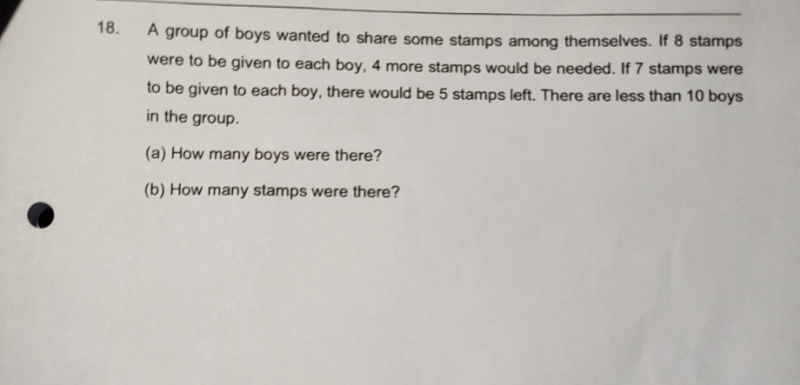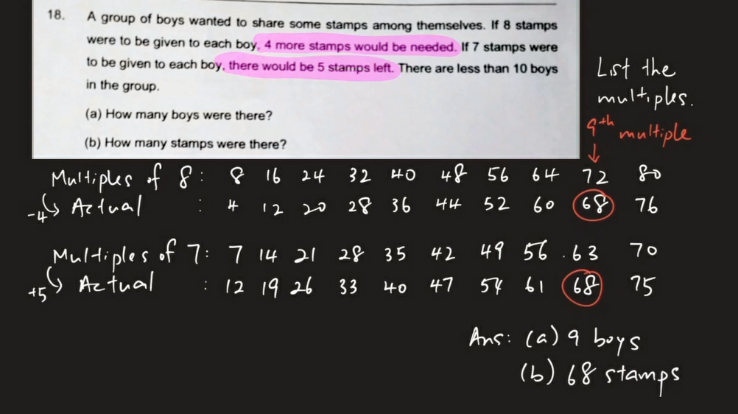# Question(a) Difference in the stamps = 4+5 = 9

Find the pair of multiples of 7 and 8 with a difference of 9.

8-7= 1,  16-14 = 2, 24-21=3…..72-63=9

Hence there are 9 boys.

(b) 72 stamps in excess of 4 stamps => 72-4 = 68

63 stamps short of 5 stamps => 63+5 = 68

Hence number of stamps is 68

0 Replies 1 LikeMultiples question.

Video Solution can be viewed here https://youtu.be/8onLBo-peKE

0 Replies 1 Like

(a)

If there are L boys ,

Then total stamps = 8L-4 = 7L+5

L=9

(b)

So there are 7*9+5=68 stamps.

0 Replies 1 Like
```Give the boys 7 stamps each. There are 5 extra left over.
Give each of the 5 extra to the first 5 boys, so they have 8 stamps each.
The question says we are now short of 4 stamps to make everyone own 8 stamps.
That means 4 boys were not given their 8-th stamps.

a. Number of boys = 5 (those who got the 8-th stamps) + 4 (those who didn't)
= 9

b. There were 9 x 7 + 5 = 68 stamps.

CHECK: 68 = 9 x 7 + 5 = 9 x 8 - 4

1234567 8
XXXXXXX .
XXXXXXX .
XXXXXXX .
XXXXXXX .
XXXXXXX X
XXXXXXX X
XXXXXXX X
XXXXXXX X
XXXXXXX X

Because 7 stamps and 8 stamps differ by 1, we can use this simple
'story' to figure it out. :-)
```